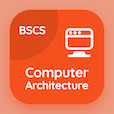Class 9 Online Courses

Grade 9 Math MCQs PDF - Topics

# Surds and Applications MCQ Quiz Online

Practice Surds and applications Multiple Choice Questions (MCQ) to solve surds and applications quiz answers PDF to learn 9th grade math online course. Algebraic Expressions and Algebraic Formulas Multiple Choice Questions and Answers (MCQs), Surds and Applications quiz questions PDF for virtual secondary school. "Surds and Applications MCQ" PDF Book: algebraic expressions, rationalization of surds test prep for online schools.

"Every surd is a/an" Multiple Choice Questions (MCQ) on surds and applications with choices irrational number, rational number, equation, and coefficient for virtual secondary school. Learn algebraic expressions and algebraic formulas quiz questions for online certificate programs for online secondary school classes.

## MCQs on Surds and Applications

MCQ: Every surd is a/an

irrational number
rational number
equation
coefficient

equation
sentence
formula
surd

MCQ: The simplest form of the surd √18 ⁄ √3 √2 is

3
√&minus3
√5
√3

MCQ: The simplest form of the surd √180 is

6√3
6√5
6
5

MCQ: The surds to be added or subtracted should be

a fraction
non-similar
similar
none of above

### More Topics from Grade 9 Math Course